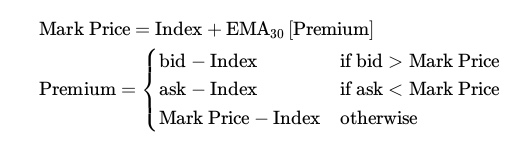# How is the mark price of futures calculated

The mark price of futures consists of the index and a 30 second exponential moving average (EMA30) of the premium, updated approximately every second. The premium is the difference between the index and the constrained last market price.The EMA30 is a smooth exponential time-based decay, where each additional second contributes 2/31, so that the centre of mass is equal to that of a standard moving average over 30 seconds.

<% if (items.length > 1) { %>